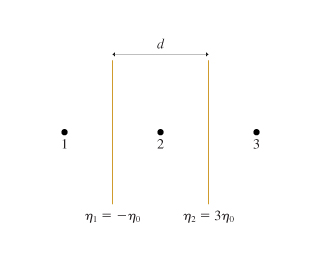# Problem: You've hung two very large sheets of plastic facing each other with distance d between them, as shown in the figure (Figure 1). By rubbing them with wool and silk, you've managed to give one sheet a uniform surface charge density η1 = −η0 and the other a uniform surface charge density η2 = +3η0.Part AWhat is the electric field vector at point 1?In the space provided, enter the numerical factor that multiplies η0ϵ0 in your answer.Part BWhat is the electric field vector at point 2?In the space provided, enter the numerical factor that multiplies η0ϵ0 in your answer.Part CWhat is the electric field vector at point 3?In the space provided, enter the numerical factor that multiplies η0ϵ0 in your answer.

###### FREE Expert Solution

Electric field:

$\overline{){\mathbf{E}}{\mathbf{=}}\frac{\mathbf{\eta }}{\mathbf{2}{\mathbf{\epsilon }}_{\mathbf{0}}}}$

Electric field lines point away from positive charges and towards negative charges.

Electric field lines point towards η1 and away from η2

87% (304 ratings)###### Problem Details

You've hung two very large sheets of plastic facing each other with distance d between them, as shown in the figure (Figure 1). By rubbing them with wool and silk, you've managed to give one sheet a uniform surface charge density η1 = −η0 and the other a uniform surface charge density η2 = +3η0.

Part A

What is the electric field vector at point 1?

In the space provided, enter the numerical factor that multiplies η0ϵ0 in your answer.

Part B

What is the electric field vector at point 2?

In the space provided, enter the numerical factor that multiplies η0ϵ0 in your answer.

Part C

What is the electric field vector at point 3?

In the space provided, enter the numerical factor that multiplies η0ϵ0 in your answer.